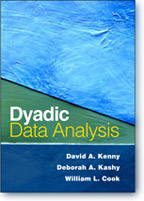May 15, 2016Chapter 2

Corrections

On page 29, in the Fisher's z transformation near the bottom of page, the letter "l" (el) in the numerator and the denominator, should be a "1" (one).

On page 44, the last sentence in the second full paragraph should end as "is increased from 1.9845 to only 1.9908, a trial increase."

Clarifications

See the webinar on nonindependence.

Elaborations

According to Landis and Koch (Landis J. R., & Koch G. (1977). The measurement of observer agreement for categorical data. Biometrics, 33, 159-174), the following standards are used to interpret kappa:

Kappa               Strength of agreement
< 0.2                 Poor
> 0.2 to 0.4        Fair
> 0.4 to 0.6        Moderate
> 0.6 to 0.8        Good
> 0.8 to 1           Very good

SPSS syntax files to compute various measures of nonindependence (inter1.sps: r, ICC, and r pairwise and inter2.sps and inter3.sps: kappa) and example data for kappa from a paper by Alferes and Kenny (2009) can be downloaded.

Methods for correcting for bias in the ICC are discussed in Donoghue, J. R., & Collins, L. M. (1990). A note on the unbiased estimation of the intraclass correlation. Psychometrika, 55, 159-164.

A better method for computing the confidence interval for the ICC is given in Cappelleri, J. C., & Ting, N. (2003). A modified large sample approach to approximate interval estimation for a particular intraclass correlation coefficient. Statistics in Medicine, 22, 1861-1877.

Shrout and Fleiss (Shrout, P., & Fleiss, J. (1979). Intraclass correlations: Uses in assessing rater reliability. Psychological Bulletin, 86, 420-428) refer to six different types of intraclass correlations. The one typically used in dyadic research is ICC(1,1).  The first "1" means each dyad has different members.  The second "1" means that the interest is in a single score.  If we wanted to average the two scores to compute a couple score, its relatability would be ICC/(ICC + 1 - ICCC/2) and would be denoted as ICC(1,2).

Data and Files

SPSS data files (sav) for the data in Table 2.1 and Table 2.3 can be downloaded.

An Excel file that can be used to test r, rI, and rP for statistical as well as compute the 95% confidence interval: ci_tests.xls.

The SPSS syntax and data file in Table 2.4 to compute kappa and it standard error: data and syntax

There are several websites that can be used to compute kappa and its standard error. One such site is Lowry at Vassar and it does also provide the 95% confidence interval. Note that it gives the standard error as .0539 whereas we obtain .0537. We are not sure why there is a difference. Perhaps Lowry uses N - 1 and not N in the formula?